Unit Rates Math Video for Kids - 6th, 7th, & 8th Grades
1%
It was processed successfully!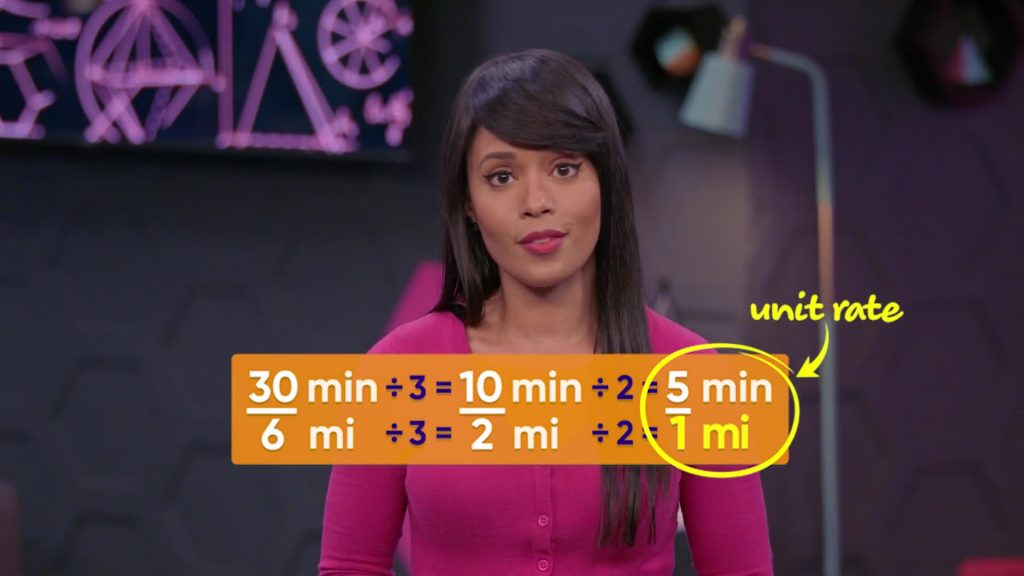WHAT ARE UNIT RATES?

A unit rate is a ratio with denominator 1. The speed of a car in miles per hour is an example of a unit rate.

To better understand unit rates…

WHAT ARE UNIT RATES?. A unit rate is a ratio with denominator 1. The speed of a car in miles per hour is an example of a unit rate. To better understand unit rates…

## LET’S BREAK IT DOWN!

### Calculate the Speed of Go-karts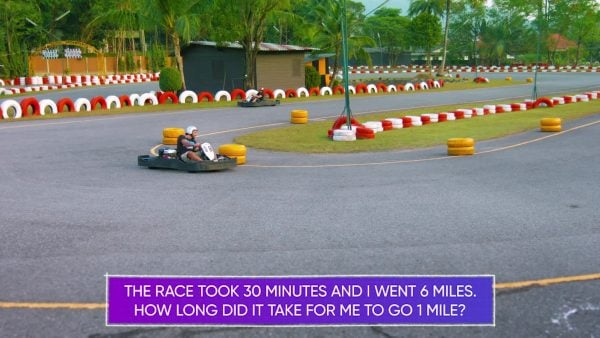Let’s say you drove a go-kart 6 miles in 30 minutes. This is a ratio of 6 miles to 30 minutes. You want to find out how much time it takes you to drive 1 mile. You can find equivalent ratios to figure this out. To find equivalent ratios, multiply or divide both the numerator and denominator in the ratio by the same number. If you divide both numbers in the ratio [ggfrac]30/6[/ggfrac] by 3, you get the equivalent ratio [ggfrac]10/2[/ggfrac]. Divide 2 again to get the ratio [ggfrac]5/1[/ggfrac]. It takes 5 minutes to drive 1 mile, or you drive at a rate of 5 minutes per mile. This ratio is called the unit rate because it has 1 in the denominator. Try this one for yourself. What is the unit rate if you drove a go-kart 9 miles in 36 minutes?

Calculate the Speed of Go-karts Let’s say you drove a go-kart 6 miles in 30 minutes. This is a ratio of 6 miles to 30 minutes. You want to find out how much time it takes you to drive 1 mile. You can find equivalent ratios to figure this out. To find equivalent ratios, multiply or divide both the numerator and denominator in the ratio by the same number. If you divide both numbers in the ratio [ggfrac]30/6[/ggfrac] by 3, you get the equivalent ratio [ggfrac]10/2[/ggfrac]. Divide 2 again to get the ratio [ggfrac]5/1[/ggfrac]. It takes 5 minutes to drive 1 mile, or you drive at a rate of 5 minutes per mile. This ratio is called the unit rate because it has 1 in the denominator. Try this one for yourself. What is the unit rate if you drove a go-kart 9 miles in 36 minutes?

### Tacos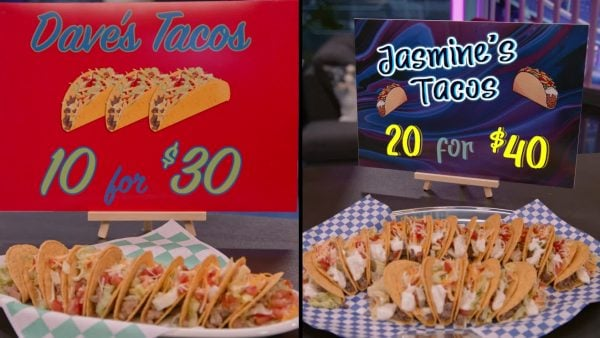Let’s say you want to buy some tacos. Dave’s Tacos offers 10 tacos for \$30. Jasmine’s Tacos offers 20 tacos for \$40. You want to find which is the better deal. Jasmine’s tacos cost \$40 for 20 tacos. You can divide by 10 and then by 2 to find a ratio of \$2 for 1 taco. You could also divide the numerator and denominator by 20 to get the unit rate of \$2 for 1 taco. This is a more efficient way to find the unit rate. Dave's deal is \$30 for 10 tacos. Divide by 10 to find that it costs \$3 to buy one of Dave's tacos. The best deal is Jasmine’s tacos because \$2 per taco is a better deal than \$3 per taco. Try this one for yourself. Mita sells 5 hamburgers for \$25. Jenell sells 12 hamburgers for \$72. Who has a better deal?

Tacos Let’s say you want to buy some tacos. Dave’s Tacos offers 10 tacos for \$30. Jasmine’s Tacos offers 20 tacos for \$40. You want to find which is the better deal. Jasmine’s tacos cost \$40 for 20 tacos. You can divide by 10 and then by 2 to find a ratio of \$2 for 1 taco. You could also divide the numerator and denominator by 20 to get the unit rate of \$2 for 1 taco. This is a more efficient way to find the unit rate. Dave's deal is \$30 for 10 tacos. Divide by 10 to find that it costs \$3 to buy one of Dave's tacos. The best deal is Jasmine’s tacos because \$2 per taco is a better deal than \$3 per taco. Try this one for yourself. Mita sells 5 hamburgers for \$25. Jenell sells 12 hamburgers for \$72. Who has a better deal?

### Dog Treats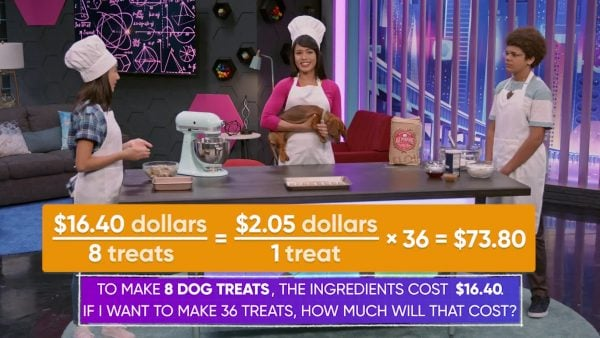Let’s say you want to make 36 dog treats. It costs \$16.40 for the ingredients to make 8 dog treats. To find out how much it costs for the ingredients to make 36 dog treats, you can first find the unit rate. Since it cost \$16.40 for the ingredients for 8 dog treats, you can divide \$16.40 by 8 to get \$2.05 per dog treat. Now that you know the cost for 1 treat, you can multiply \$2.05 by 36 to find the cost for 36. \$2.05 × 36 = \$73.80. It costs \$73.80 to make 36 dog treats. Try this one for yourself. It costs \$54 to make 12 cookies. How much does it cost to make 18 cookies?

Dog Treats Let’s say you want to make 36 dog treats. It costs \$16.40 for the ingredients to make 8 dog treats. To find out how much it costs for the ingredients to make 36 dog treats, you can first find the unit rate. Since it cost \$16.40 for the ingredients for 8 dog treats, you can divide \$16.40 by 8 to get \$2.05 per dog treat. Now that you know the cost for 1 treat, you can multiply \$2.05 by 36 to find the cost for 36. \$2.05 × 36 = \$73.80. It costs \$73.80 to make 36 dog treats. Try this one for yourself. It costs \$54 to make 12 cookies. How much does it cost to make 18 cookies?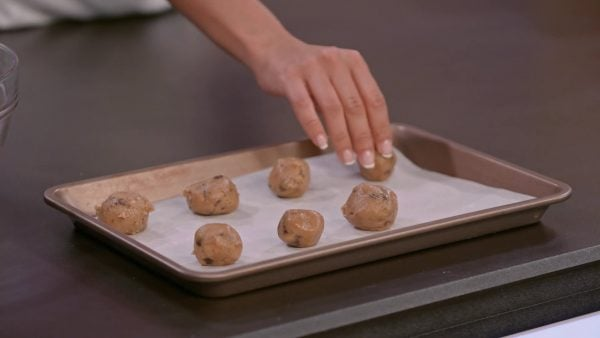You want to use Adesina’s World Famous Chocolate Chip Cookie Recipe to make some cookies. You need 1[ggfrac]1/2[/ggfrac] cups of sugar for every [ggfrac]2/5[/ggfrac] cup of chocolate chips. You have 10 cups of chocolate chips to use. If you want to use all of the chips, how much sugar do you need? First, find how much sugar you need for 1 cup of chips. The ratio of sugar to chips is 1[ggfrac]1/2[/ggfrac] to [ggfrac]2/5[/ggfrac]. To find the unit rate, divide 1[ggfrac]1/2[/ggfrac] by [ggfrac]2/5[/ggfrac]. [ggfrac]3/2[/ggfrac] ÷ [ggfrac]2/5[/ggfrac] = [ggfrac]1/2[/ggfrac] × [ggfrac]5/2[/ggfrac] = [ggfrac]15/4[/ggfrac] = 3[ggfrac]3/4[/ggfrac]. The unit rate is 3[ggfrac]3/4[/ggfrac] cups of sugar per 1 cup of chips. To use all 10 cups of chocolate chips, multiply 3[ggfrac]3/4[/ggfrac] by 10 to find the amount of sugar you need. 10 × 3[ggfrac]3/4[/ggfrac] = 10 × [ggfrac]15/4[/ggfrac] = [ggfrac]150/4[/ggfrac] = 37[ggfrac]1/2[/ggfrac]. You need 37[ggfrac]1/2[/ggfrac] cups of sugar. Try this one for yourself. For a cake recipe you need 2 [ggfrac]1/4[/ggfrac] cups of milk. If you have 15 cups of milk and want to use it all to make cakes, how many cups of flour do you need?

Chocolate Chip Cookie Recipe You want to use Adesina’s World Famous Chocolate Chip Cookie Recipe to make some cookies. You need 1[ggfrac]1/2[/ggfrac] cups of sugar for every [ggfrac]2/5[/ggfrac] cup of chocolate chips. You have 10 cups of chocolate chips to use. If you want to use all of the chips, how much sugar do you need? First, find how much sugar you need for 1 cup of chips. The ratio of sugar to chips is 1[ggfrac]1/2[/ggfrac] to [ggfrac]2/5[/ggfrac]. To find the unit rate, divide 1[ggfrac]1/2[/ggfrac] by [ggfrac]2/5[/ggfrac]. [ggfrac]3/2[/ggfrac] ÷ [ggfrac]2/5[/ggfrac] = [ggfrac]1/2[/ggfrac] × [ggfrac]5/2[/ggfrac] = [ggfrac]15/4[/ggfrac] = 3[ggfrac]3/4[/ggfrac]. The unit rate is 3[ggfrac]3/4[/ggfrac] cups of sugar per 1 cup of chips. To use all 10 cups of chocolate chips, multiply 3[ggfrac]3/4[/ggfrac] by 10 to find the amount of sugar you need. 10 × 3[ggfrac]3/4[/ggfrac] = 10 × [ggfrac]15/4[/ggfrac] = [ggfrac]150/4[/ggfrac] = 37[ggfrac]1/2[/ggfrac]. You need 37[ggfrac]1/2[/ggfrac] cups of sugar. Try this one for yourself. For a cake recipe you need 2 [ggfrac]1/4[/ggfrac] cups of milk. If you have 15 cups of milk and want to use it all to make cakes, how many cups of flour do you need?

## UNIT RATE VOCABULARY

Rate
A quantity measured with respect to another quantity.
Fraction
A fraction shows how many parts of a whole you have.
Denominator
The bottom number in a fraction. Shows how many parts are in the whole.
Numerator
The top number in a fraction. Shows how many parts of the whole you have.
Unit rate
A ratio with denominator 1.
Ratio
A comparison between two quantities.
Ratios are equivalent if one can be expressed as a multiple of the other.
A table that shows equivalent ratios.

## UNIT RATE DISCUSSION QUESTIONS

### There are 36 people in 9 cars. There is the same number of people in each car. How can you use equivalent ratios to find the unit rate?

The rate is 36 people per 9 cars, or [ggfrac]36/9[/ggfrac].

[ggfrac]36/9[/ggfrac] = [ggfrac]12/3[/ggfrac] = [ggfrac]4/1[/ggfrac]

The unit rate is 4 people per car.

### Elton bought 7 shirts for \$66.50. What is the unit rate of cost per t-shirt? How did you find the unit rate?

The unit rate is \$9.50 per shirt. I found the unit rate by dividing \$66.50 by 7.

### At Tala’s Sporting Goods Store, 6 baseballs cost \$33. At Osher’s Sporting Goods Store, 4 baseballs cost \$24. How can you use unit rates to show which store has a better deal on baseballs?

Tala’s Sporting Goods Store:

[ggfrac]\$33/6[/ggfrac] = [ggfrac]\$5.50/1[/ggfrac] The unit rate is \$5.50 per baseball.

Osher's Sporting Goods Store:

[ggfrac]\$24/4[/ggfrac] = [ggfrac]\$6/1[/ggfrac] The unit rate is \$6.00 per baseball.

Tala’s Sporting Goods Store has a better deal.

### Marcos works 36 hours in 8 days. How many hours does he work in 20 days? How can you use unit rates to solve this problem?

[ggfrac]36/8[/ggfrac] = [ggfrac]4.5/1[/ggfrac], so the unit rate is 4.5 hours per day.

4.5 × 20 = 90. Marcos works 90 hours in 20 days.

### For Sandra’s favorite cookies, she uses 1[ggfrac]1/2[/ggfrac] cups of sugar for every 2[ggfrac]1/4[/ggfrac] cups of flour. She wants to make as many cookies as she can with 9 cups of sugar. Use a unit rate to find how much flour she needs to make the cookies.

Rate of flour to sugar: 2[ggfrac]1/4[/ggfrac] / 1[ggfrac]1/2[/ggfrac]

Unit rate of flour to sugar:

2[ggfrac]1/4[/ggfrac] / 1[ggfrac]1/2[/ggfrac] = [ggfrac]9/4[/ggfrac] x [ggfrac]2/3[/ggfrac] = [ggfrac]18/22[/ggfrac] = [ggfrac]3/2[/ggfrac] cups of flour for every cup of sugar

Multiply [ggfrac]3/2[/ggfrac] × 9 to find out how much flour she needs:

[ggfrac]3/2[/ggfrac] x 9 = 13[ggfrac]1/2[/ggfrac] cups of flour

Sandra needs 13[ggfrac]1/2[/ggfrac] cups of flour to make the cookies with 9 cups of sugar.
X

## Success

We’ve sent you an email with instructions how to reset your password.
Ok